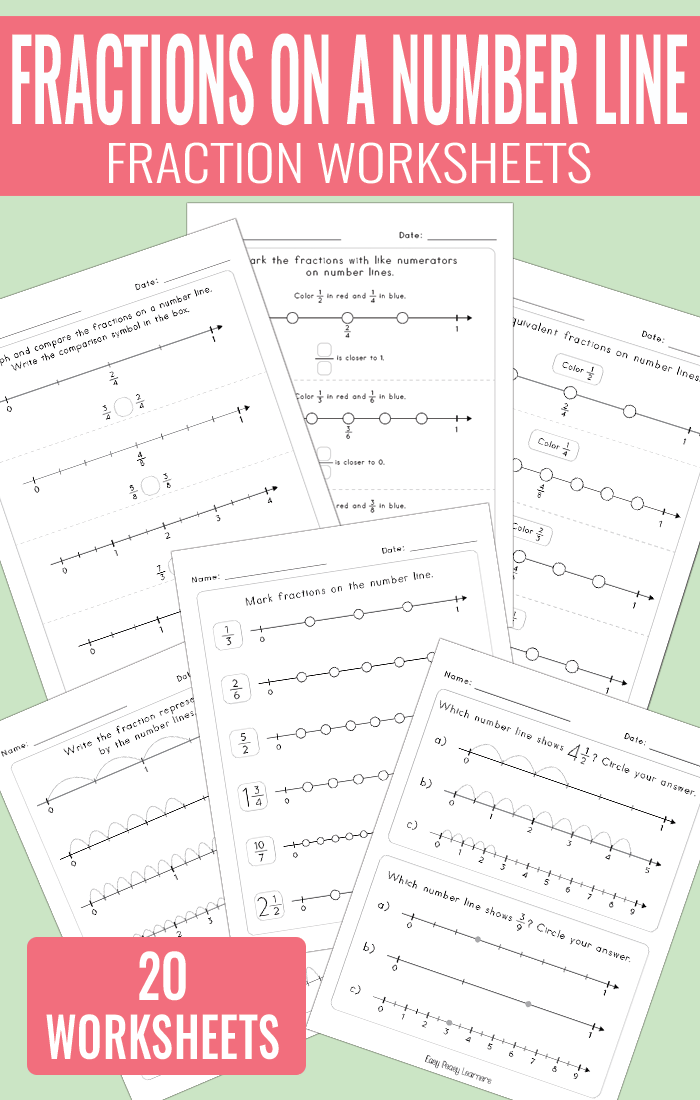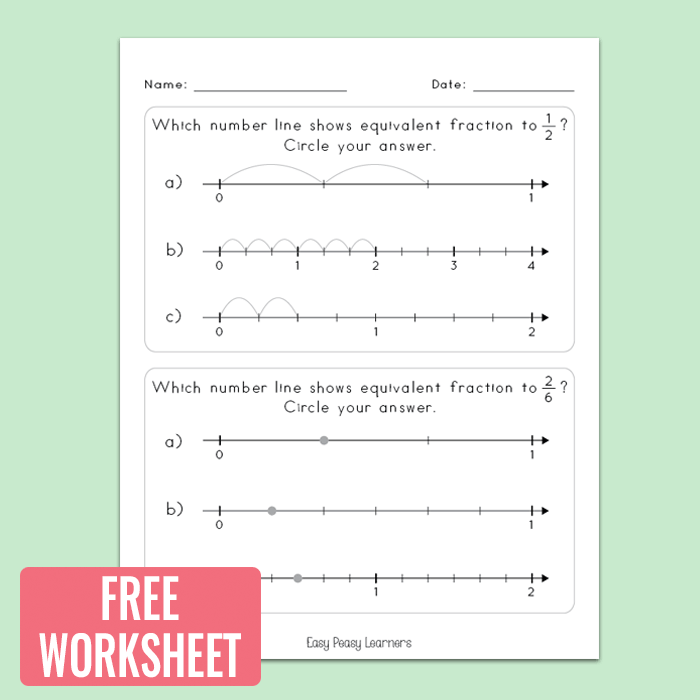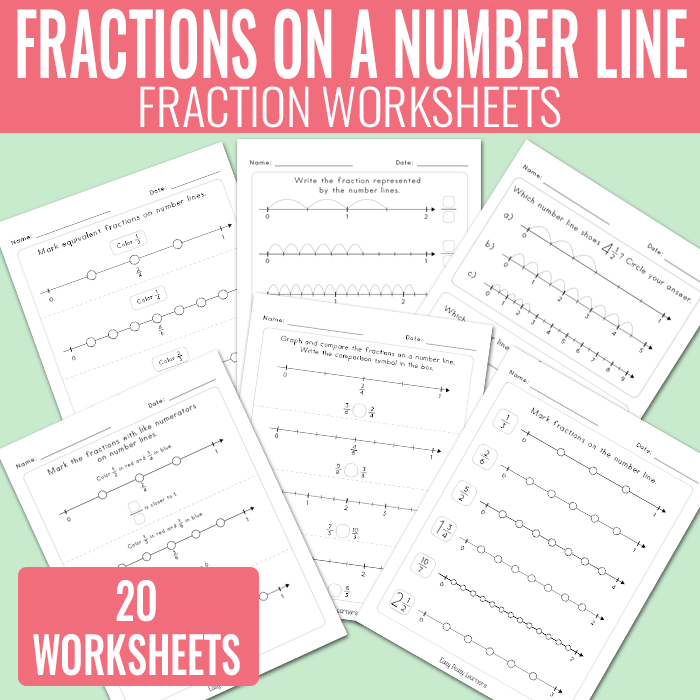# Fractions on a Number Line Worksheets – Math Worksheets

Fractions are a tough subject for students to master so this is one of that areas that requires a lot of practice. This set of Fractions on a number line worksheets focuses on both marking the fractions on a number line and reading them from the line.## Fractions on a Number Line Worksheets

There are 20 different worksheets in this printable worksheet set

• it includes 20 unique addition and subtraction up to 10 worksheets
• different difficulty levels, you can start with simpler exercises – the ones that require the students to circle the answers and continue with harder ones.

You can get the whole set at Teachers Pay Teachers where you can also preview the whole set.

Get Fractions on a Number Line Printable Worksheets

Get Fractions on a Number Lines Worksheets

These worksheets can be laminated for continuous use.Want to give these a try? You can grab one of the pages for free

Grab the fractions on a number line free worksheet freebie worksheet

Get Fractions on a Number Line Printable Worksheets

Get Fractions on a Number Lines Worksheets

More Fractions Worksheets

Equivalent Fractions Worksheets